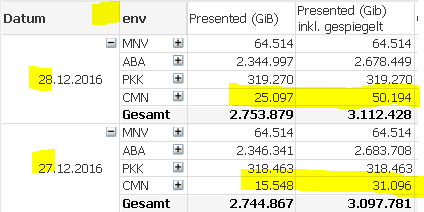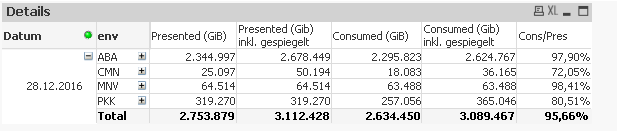# QlikView App Dev

Discussion Board for collaboration related to QlikView App Development.

Announcements
Skip the ticket, Chat with Qlik Support instead for instant assistance.
cancel
Showing results for
Did you mean:Not applicable

Dear Community,

I'm pretty new to qlik and now I have a problem that despite all my efforts I can't get solved without your help.

Here is the description:

1. Users can enter the number of days they want to see. This creates the time period within the variable vZeitraumSelektion

2. Under certain conditions, the results must be multiplied by 2this is my expression:

= sum({<\$(vZeitraumSelektion), Usage_Flag={'presented_gib'}>} Wert)
+ sum({<
\$(vZeitraumSelektion)>}
aggr({<
\$(vZeitraumSelektion)>}
if(right(pool,1)='M' and env<>'EMC' and env<>'PKK',
sum({\$<
\$(vZeitraumSelektion), Usage_Flag={'presented_gib'}>} Wert),
0),
lun, pool, env, Kunde, Datum))

3. This works fine, until there is a filter on the date, In this case, only the last day will be calculated.The intention is to calculate all days (vZeitraumSelektion) up to the max selekted date. What am I doing wrong here?

Just in case, the qvw is attached:

Many thanks in advance for every hint.

1 Solution

Accepted SolutionsNot applicable
Author

Solved 😄

In the event that someone wants to achieve something similar.

Values ​​should be doubled if the following is true:

If (right (pool, 1) = 'M' and env <> 'EMC' and env <> 'PKK'

Instead of placing the if-function within the aggr, I turned it around. So this is, what works for me:

=sum({<\$(vZeitraumSelektion)>} if(right(pool,1)='M' and env<>'EMC' and env<>'PKK',
aggr(sum({<
\$(vZeitraumSelektion), Usage_Flag={'presented_gib'}>} Wert)*2, lun, pool, env, Kunde, Datum),
aggr(sum({<
\$(vZeitraumSelektion), Usage_Flag={'presented_gib'}>} Wert), lun, pool, env, Kunde, Datum)))

Thank all of you very much

5 RepliesMVP

Are you looking to get this?Not applicable
Author

No. The idea is, to show a certain amount of days up to a specific date. If for example the 27.12. is selected and the variable vZeitraumSelektion is equal 7, then 7 days until 27.12. are displayed.

The problem in this case is the calculation in Presented (GiB). It is only working for the first day. Every following day don't get the additional counts, they should have.

Basically, the counts should look like the first screen shot when the 28. is selected. Hope this makes sense.MVP

I don't think I understand still. Would you be able to provide the numbers you expect to see when 27.12 is selected?Creator II

Hello,

so the Filter in "Datum" should not be done?

Have a look here:

https://help.qlik.com/de-DE/qlikview/12.0/Subsystems/Client/Content/ChartFunctions/SetAnalysis/set-a...

Hallo,

soll der Filter im Datum ignoriert werden?

Vielleicht bringt dich das in die richtige Richtung!

SGNot applicable
Author

Solved 😄

In the event that someone wants to achieve something similar.

Values ​​should be doubled if the following is true:

If (right (pool, 1) = 'M' and env <> 'EMC' and env <> 'PKK'

Instead of placing the if-function within the aggr, I turned it around. So this is, what works for me:

=sum({<\$(vZeitraumSelektion)>} if(right(pool,1)='M' and env<>'EMC' and env<>'PKK',
aggr(sum({<
\$(vZeitraumSelektion), Usage_Flag={'presented_gib'}>} Wert)*2, lun, pool, env, Kunde, Datum),
aggr(sum({<
\$(vZeitraumSelektion), Usage_Flag={'presented_gib'}>} Wert), lun, pool, env, Kunde, Datum)))

Thank all of you very muchCommunity Browser